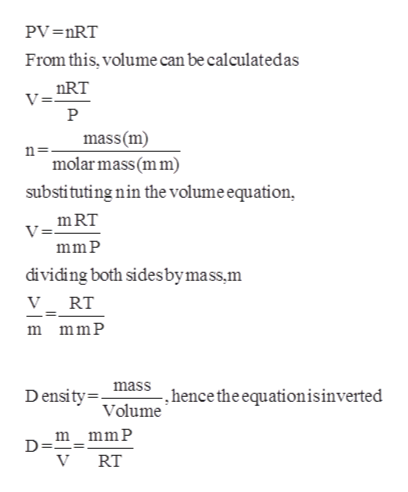# What is the density of mustard gas, C4H8Cl2S, at 265 mmHg and a temperature of 315K

Question
44 views

What is the density of mustard gas, C4H8Cl2S, at 265 mmHg and a temperature of 315K

check_circle

Step 1

Given,

Pressure = 265mmHg.

Temperature = 315K.

Density is the ratio of mass and vol...help_outlineImage TranscriptionclosePV=nRT From this, volume can be calculated as nRT V= P mass (m) molar mass (mm) n= substituting nin the volume equation, mRT V= mm P dividing both sidesbymass,m V_ RT m mmP mass Density -, hence the equationisinverted Volume m_mmP D = V RT fullscreen

### Want to see the full answer?

See Solution

#### Want to see this answer and more?

Solutions are written by subject experts who are available 24/7. Questions are typically answered within 1 hour.*

See Solution
*Response times may vary by subject and question.
Tagged in

### Physical Chemistry